|

# 轻奢生活,从拥有一个恒洁Q10智能一体机开始

提到马桶，大多数人首先会想到日本。这个注重品质和细节的国家，有着科技感十足的马桶，智能马桶盖的普及率全球第一。但第一个抽水马桶却由文艺复兴时期的英格兰诗人——约翰·哈林顿，在 1594 年发明，共制造了两个，其中一个的主人就是英国女王伊丽莎白一世。而在今天，马桶已不再是贵族的专利，普通大众可以轻易买到心仪的马桶。随着不断的改良，产品线繁杂，结构功能外观各式各样，选择马桶时的你是否有种如同便秘的痛苦？

现如今，恒洁Q10智能一体机全新上市，让智能马桶在拥有华丽外观的同时也拥有强硬的内核。下面小狐就来为大家展示在挑选马桶时应该注意到的那些方面，彻底治好大家的纠结症。

智感即冲让生活感性充盈

高智商，智能微波感应科技，站与坐皆能自动识别，让您忘记冲水这件事。将“男女平等”充分体现，利用智能微波感应科技，无论男生女生使用后它都能自动识别，即时冲水，高智商、高情商，全面解放全家人的双手，舒享洁净空间。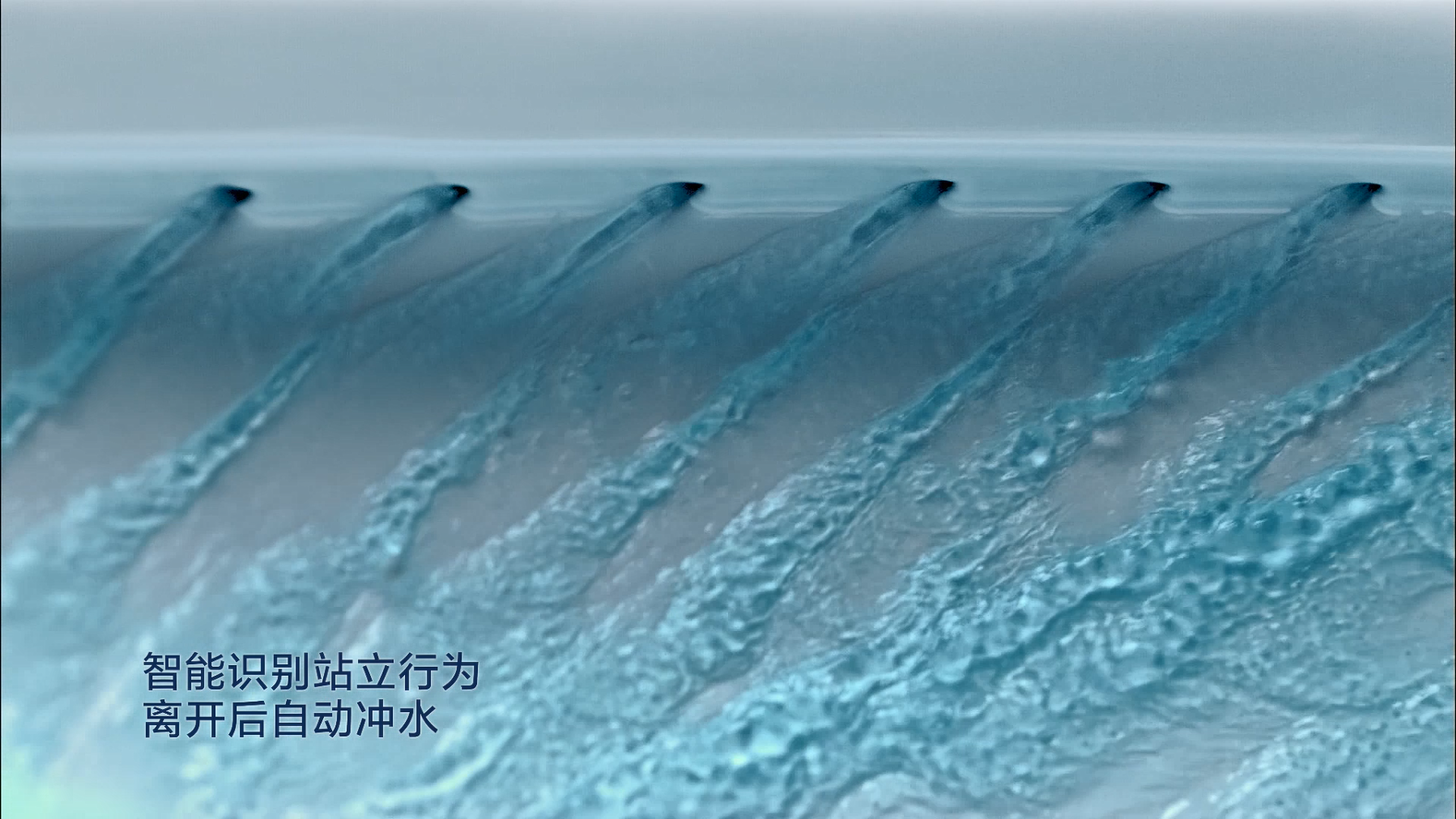靓眼视窗展现人机互动

有华丽优质的外表，标配是出众富涵的内核，恒洁Q10智能一体机设计了靓眼LED视窗，外观设计更是前卫大气，以汽车仪表盘为灵感，看起来科技感十足，重要参数也一目了然，，线条简洁流畅，显示屏部分以跑车仪表盘为灵感。另外增设了智慧眼视窗平台，Led互动设计，靠近时自动点亮，更具人机互动感赋予Q10 更多动感。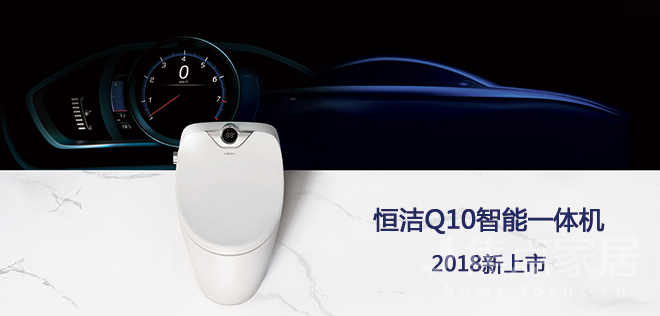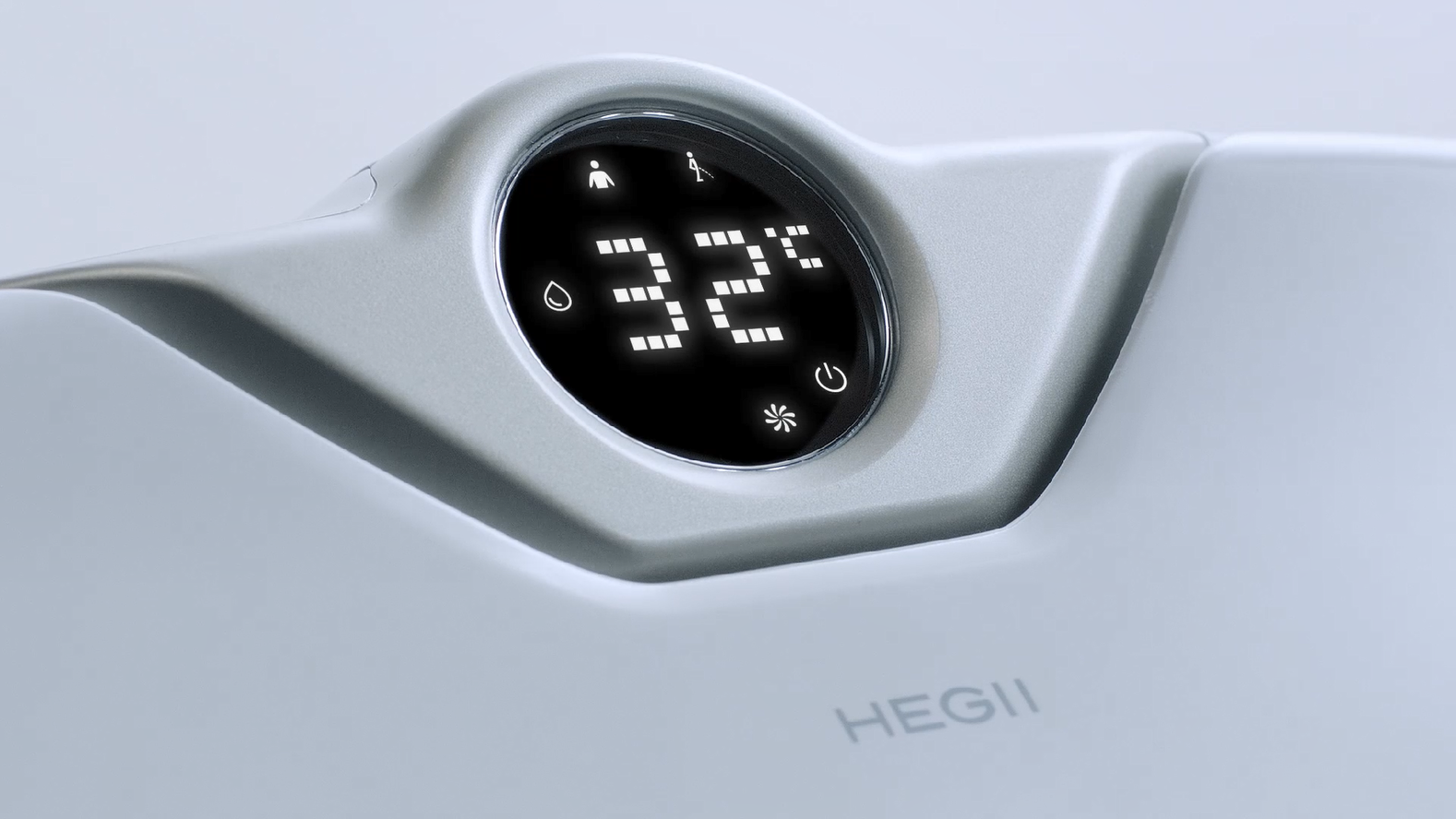“荷叶疏水”，“银离子抗菌”材质，保驾护航

马桶作为细菌最容易滋生的地方，其釉面清洁和排污力度也是消费者最关注的地方。细菌最容易从这些部位进入体内，而如厕用水后彻底清洁，可以降低细菌的滋生，减少妇科病和肛肠类疾病的患病几率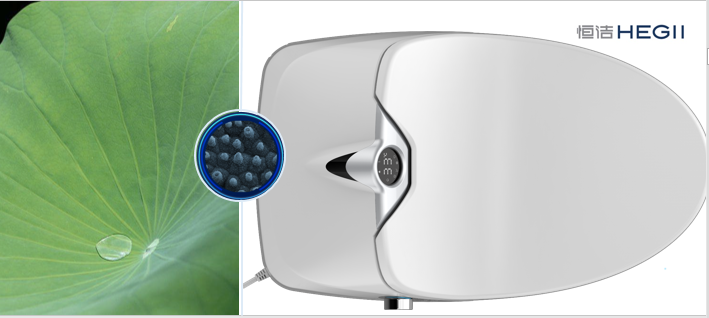Q10的新增功能还体现在翻盖的材质使用了荷叶疏水材料运用仿生科技，在表面构筑独特的微纳米结构，快速将水渍分解成小水珠并在倾斜时滑落，实现抗污易洁的效果。银离子抗菌座圈添加符合日本SIAA（抗菌产品技术协议会）认证的纳米银离子材料，带来长效稳定的抗菌效果。能够有效抑制细菌繁殖。

智能翻盖带来极致未来体验

厌烦了千篇一律的翻盖，弯腰再弯腰，那就来点不一样的，高智商的恒洁Q10察觉到了这一点，并加以优化，将翻盖体验做到了极致。两种模式，在能自动感应翻盖的同时，亦可选择手触摸翻盖，只需要轻轻触碰，马桶盖自动开启，带来全新体验。

男人认为，女人在上完厕所后应该把马桶圈拿起来；女人则认为，男人在上完厕所后应该把马桶圈放下来。Q10可以自动感应翻盖，在使用过程中，马桶盖会自动缓缓闭合和开启，不用手去掀开盖上，减少手部细菌接触。也可以选择手触翻盖模式，按下盖板左侧按键会翻开一个座盖，按下右侧则会翻开两个座盖。老人上了年纪之后行动不便、视力下降，如厕也会变得艰难，所以烘干、自动感应闭合、夜灯这些功能都很适合老人。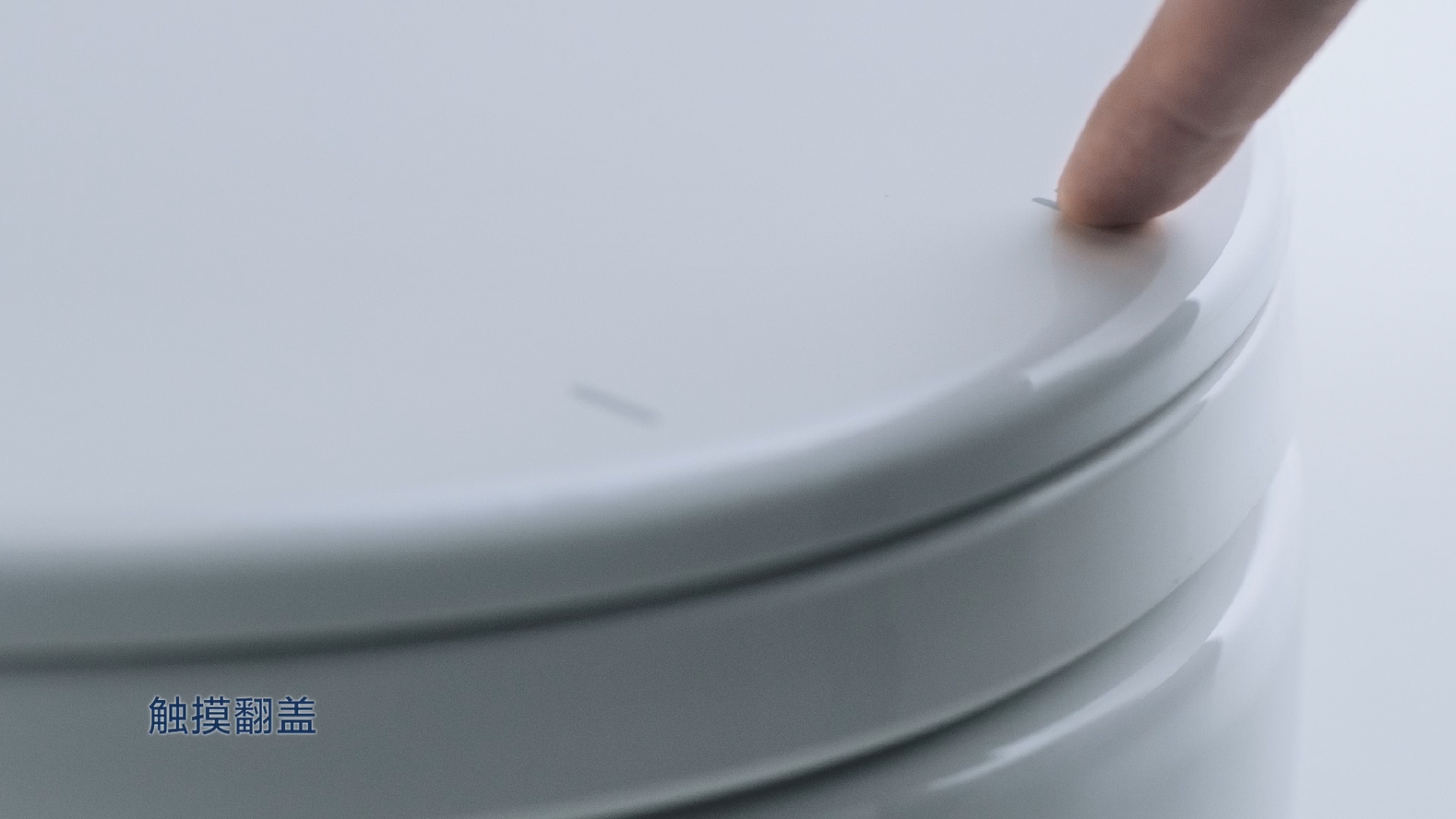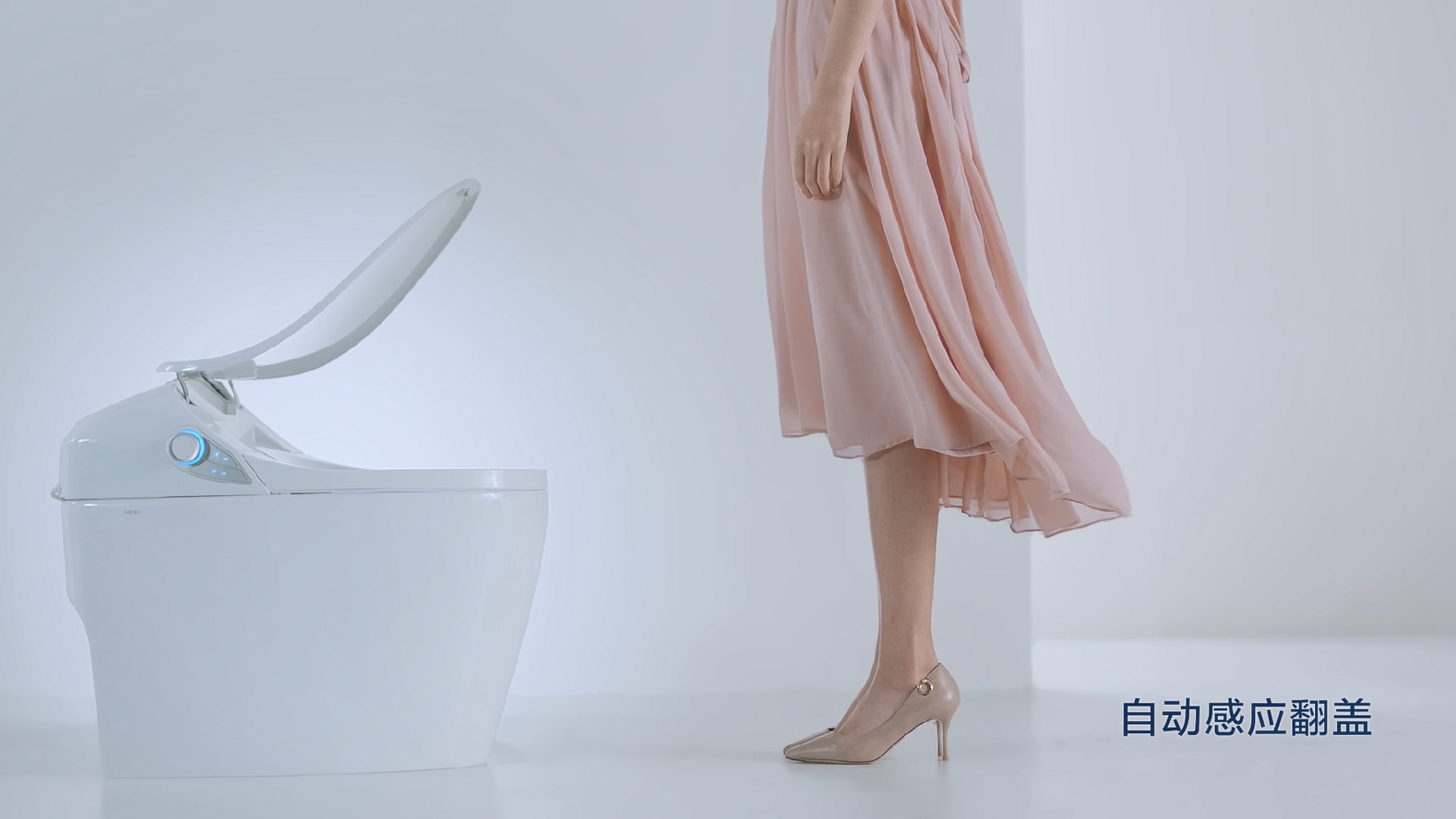恒洁Q10智能一体机拥有水漩能系统，冲厕时冲力稳定强劲，就算家里停电了或者水压很低，也不会有影响。当然，它还有很多贴心的小细节，像妇洗功能，可以在女生使用后实现自动清洗，出水的喷头和其他清洗模式也不同，喷头光洁不易挂污，水洗完成后还会帮你贴心烘干。而针对于男士的站立行为，系统能够做到自定识别甄选，因此不管是站着还是坐着如厕，都可以做到自动冲水，这样的高智商高情商的产品有没有惊艳到你。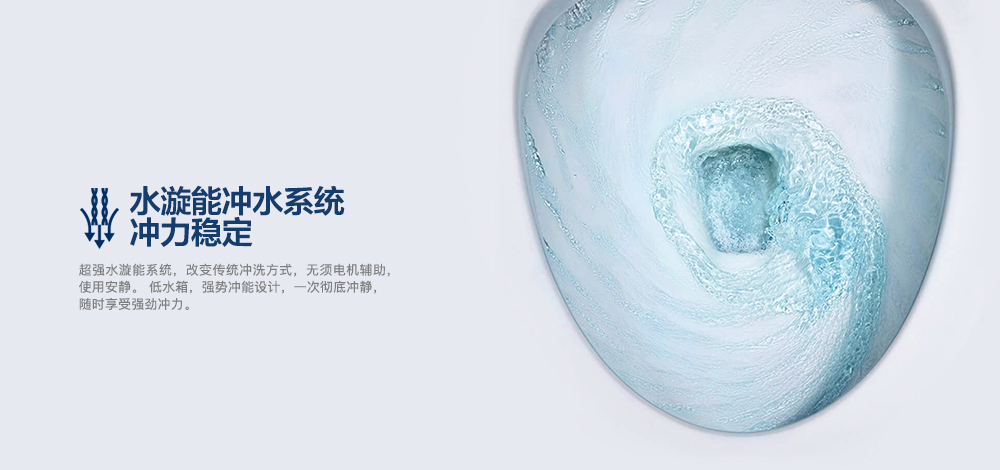让马桶的造型更精致，少了各种凹凸与线条，让马桶不再是厕所里刷存在感的那个笨重家伙。而是更好的融合在整个卫浴空间里，简洁、低调，带点大隐隐于市的禅意。产品在设计上增添了恒洁28.5°承托系统，坐感更舒适，符合亚洲人体型设计，久坐不麻，让你尽情畅享如厕空间。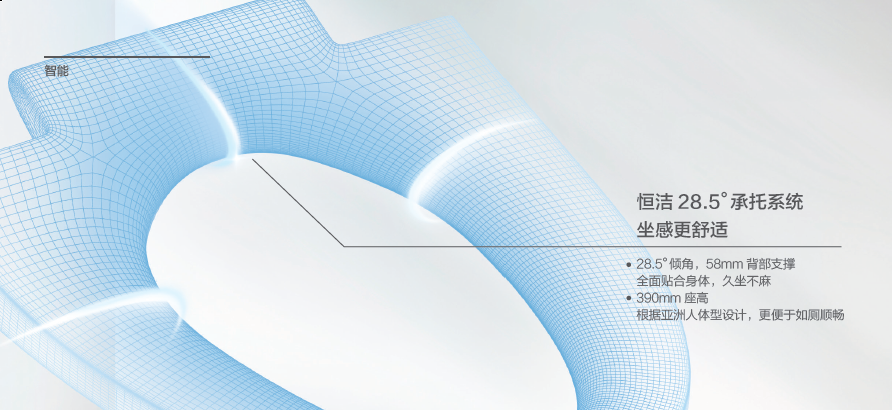活水即热瞬间加温持久恒温

这个功能对于冬天如厕的人，可谓是用一次就再也离不开。设想这样一个场景，寒冷的冬天，大半夜的从温暖的被窝里起来，睡眼惺忪的去上厕所，结果屁股一碰到马桶盖就给冰得一哆嗦，每次都要鼓足勇气才敢坐上去。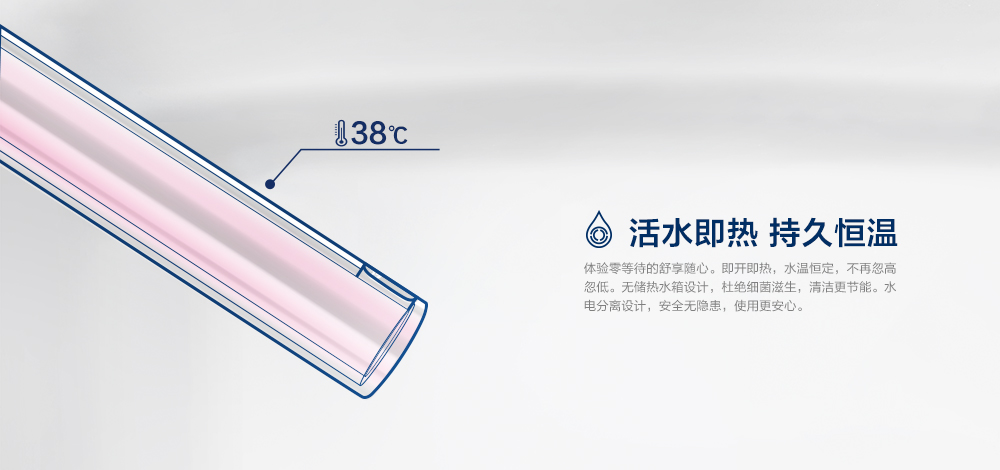所以加热座圈是非常重要的。一个好的加热座圈应该具备这样的属性，首先是温度可调，有些人喜欢温一点有些人喜欢热一点，有几档可调至少可以保证能满足大多数人的需求。但是我们在考虑舒适的时候，也要考虑到安全。恒洁Q10智能一体机，能在任何时刻加热升温，在有活水的同时瞬间加温，无需等待，并能保证持久恒温，倍感呵护你和家人的身心。

小编总结：

智能是一个普罗大众都知道的名词，但怎样将它发挥给人印象深刻，我想恒洁就很好的做到了这一点，不论从何种角度出发，它的产品都能给你带来高品质的感受。在这个大肆宣扬的智能时代，还能坚守至臻之心，这一点就有很多人是望尘莫及的，我想将智能做到恒洁这样就是坚守匠心。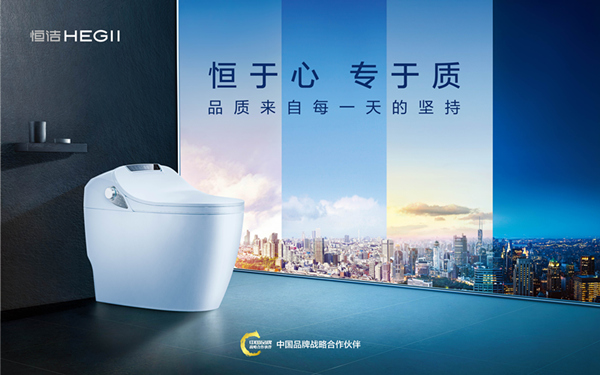`声明：本文由入驻焦点开放平台的作者撰写，除焦点官方账号外，观点仅代表作者本人，不代表焦点立场错误信息举报电话： 400-099-0099，邮箱：jubao@vip.sohu.com，或点此进行意见反馈，或点此进行举报投诉。`A B C D E F G H J K L M N P Q R S T W X Y Z
A - B - C - D - E
• A
• 鞍山
• 安庆
• 安阳
• 安顺
• 安康
• 澳门
• B
• 北京
• 保定
• 包头
• 巴彦淖尔
• 本溪
• 蚌埠
• 亳州
• 滨州
• 北海
• 百色
• 巴中
• 毕节
• 保山
• 宝鸡
• 白银
• 巴州
• C
• 承德
• 沧州
• 长治
• 赤峰
• 朝阳
• 长春
• 常州
• 滁州
• 池州
• 长沙
• 常德
• 郴州
• 潮州
• 崇左
• 重庆
• 成都
• 楚雄
• 昌都
• 慈溪
• 常熟
• D
• 大同
• 大连
• 丹东
• 大庆
• 东营
• 德州
• 东莞
• 德阳
• 达州
• 大理
• 德宏
• 定西
• 儋州
• 东平
• E
• 鄂尔多斯
• 鄂州
• 恩施
F - G - H - I - J
• F
• 抚顺
• 阜新
• 阜阳
• 福州
• 抚州
• 佛山
• 防城港
• G
• 赣州
• 广州
• 桂林
• 贵港
• 广元
• 广安
• 贵阳
• 固原
• H
• 邯郸
• 衡水
• 呼和浩特
• 呼伦贝尔
• 葫芦岛
• 哈尔滨
• 黑河
• 淮安
• 杭州
• 湖州
• 合肥
• 淮南
• 淮北
• 黄山
• 菏泽
• 鹤壁
• 黄石
• 黄冈
• 衡阳
• 怀化
• 惠州
• 河源
• 贺州
• 河池
• 海口
• 红河
• 汉中
• 海东
• 怀来
• I
• J
• 晋中
• 锦州
• 吉林
• 鸡西
• 佳木斯
• 嘉兴
• 金华
• 景德镇
• 九江
• 吉安
• 济南
• 济宁
• 焦作
• 荆门
• 荆州
• 江门
• 揭阳
• 金昌
• 酒泉
• 嘉峪关
K - L - M - N - P
• K
• 开封
• 昆明
• 昆山
• L
• 廊坊
• 临汾
• 辽阳
• 连云港
• 丽水
• 六安
• 龙岩
• 莱芜
• 临沂
• 聊城
• 洛阳
• 漯河
• 娄底
• 柳州
• 来宾
• 泸州
• 乐山
• 六盘水
• 丽江
• 临沧
• 拉萨
• 林芝
• 兰州
• 陇南
• M
• 牡丹江
• 马鞍山
• 茂名
• 梅州
• 绵阳
• 眉山
• N
• 南京
• 南通
• 宁波
• 南平
• 宁德
• 南昌
• 南阳
• 南宁
• 内江
• 南充
• P
• 盘锦
• 莆田
• 平顶山
• 濮阳
• 攀枝花
• 普洱
• 平凉
Q - R - S - T - W
• Q
• 秦皇岛
• 齐齐哈尔
• 衢州
• 泉州
• 青岛
• 清远
• 钦州
• 黔南
• 曲靖
• 庆阳
• R
• 日照
• 日喀则
• S
• 石家庄
• 沈阳
• 双鸭山
• 绥化
• 上海
• 苏州
• 宿迁
• 绍兴
• 宿州
• 三明
• 上饶
• 三门峡
• 商丘
• 十堰
• 随州
• 邵阳
• 韶关
• 深圳
• 汕头
• 汕尾
• 三亚
• 三沙
• 遂宁
• 山南
• 商洛
• 石嘴山
• T
• 天津
• 唐山
• 太原
• 通辽
• 铁岭
• 泰州
• 台州
• 铜陵
• 泰安
• 铜仁
• 铜川
• 天水
• 天门
• W
• 乌海
• 乌兰察布
• 无锡
• 温州
• 芜湖
• 潍坊
• 威海
• 武汉
• 梧州
• 渭南
• 武威
• 吴忠
• 乌鲁木齐
X - Y - Z
• X
• 邢台
• 徐州
• 宣城
• 厦门
• 新乡
• 许昌
• 信阳
• 襄阳
• 孝感
• 咸宁
• 湘潭
• 湘西
• 西双版纳
• 西安
• 咸阳
• 西宁
• 仙桃
• 西昌
• Y
• 运城
• 营口
• 盐城
• 扬州
• 鹰潭
• 宜春
• 烟台
• 宜昌
• 岳阳
• 益阳
• 永州
• 阳江
• 云浮
• 玉林
• 宜宾
• 雅安
• 玉溪
• 延安
• 榆林
• 银川
• Z
• 张家口
• 镇江
• 舟山
• 漳州
• 淄博
• 枣庄
• 郑州
• 周口
• 驻马店
• 株洲
• 张家界
• 珠海
• 湛江
• 肇庆
• 中山
• 自贡
• 资阳
• 遵义
• 昭通
• 张掖
• 中卫

1室1厅1厨1卫1阳台

1
2
3
4
5

0
1
2

1

1

0
1
2
3报名成功，资料已提交审核A B C D E F G H J K L M N P Q R S T W X Y Z
A - B - C - D - E
• A
• 鞍山
• 安庆
• 安阳
• 安顺
• 安康
• 澳门
• B
• 北京
• 保定
• 包头
• 巴彦淖尔
• 本溪
• 蚌埠
• 亳州
• 滨州
• 北海
• 百色
• 巴中
• 毕节
• 保山
• 宝鸡
• 白银
• 巴州
• C
• 承德
• 沧州
• 长治
• 赤峰
• 朝阳
• 长春
• 常州
• 滁州
• 池州
• 长沙
• 常德
• 郴州
• 潮州
• 崇左
• 重庆
• 成都
• 楚雄
• 昌都
• 慈溪
• 常熟
• D
• 大同
• 大连
• 丹东
• 大庆
• 东营
• 德州
• 东莞
• 德阳
• 达州
• 大理
• 德宏
• 定西
• 儋州
• 东平
• E
• 鄂尔多斯
• 鄂州
• 恩施
F - G - H - I - J
• F
• 抚顺
• 阜新
• 阜阳
• 福州
• 抚州
• 佛山
• 防城港
• G
• 赣州
• 广州
• 桂林
• 贵港
• 广元
• 广安
• 贵阳
• 固原
• H
• 邯郸
• 衡水
• 呼和浩特
• 呼伦贝尔
• 葫芦岛
• 哈尔滨
• 黑河
• 淮安
• 杭州
• 湖州
• 合肥
• 淮南
• 淮北
• 黄山
• 菏泽
• 鹤壁
• 黄石
• 黄冈
• 衡阳
• 怀化
• 惠州
• 河源
• 贺州
• 河池
• 海口
• 红河
• 汉中
• 海东
• 怀来
• I
• J
• 晋中
• 锦州
• 吉林
• 鸡西
• 佳木斯
• 嘉兴
• 金华
• 景德镇
• 九江
• 吉安
• 济南
• 济宁
• 焦作
• 荆门
• 荆州
• 江门
• 揭阳
• 金昌
• 酒泉
• 嘉峪关
K - L - M - N - P
• K
• 开封
• 昆明
• 昆山
• L
• 廊坊
• 临汾
• 辽阳
• 连云港
• 丽水
• 六安
• 龙岩
• 莱芜
• 临沂
• 聊城
• 洛阳
• 漯河
• 娄底
• 柳州
• 来宾
• 泸州
• 乐山
• 六盘水
• 丽江
• 临沧
• 拉萨
• 林芝
• 兰州
• 陇南
• M
• 牡丹江
• 马鞍山
• 茂名
• 梅州
• 绵阳
• 眉山
• N
• 南京
• 南通
• 宁波
• 南平
• 宁德
• 南昌
• 南阳
• 南宁
• 内江
• 南充
• P
• 盘锦
• 莆田
• 平顶山
• 濮阳
• 攀枝花
• 普洱
• 平凉
Q - R - S - T - W
• Q
• 秦皇岛
• 齐齐哈尔
• 衢州
• 泉州
• 青岛
• 清远
• 钦州
• 黔南
• 曲靖
• 庆阳
• R
• 日照
• 日喀则
• S
• 石家庄
• 沈阳
• 双鸭山
• 绥化
• 上海
• 苏州
• 宿迁
• 绍兴
• 宿州
• 三明
• 上饶
• 三门峡
• 商丘
• 十堰
• 随州
• 邵阳
• 韶关
• 深圳
• 汕头
• 汕尾
• 三亚
• 三沙
• 遂宁
• 山南
• 商洛
• 石嘴山
• T
• 天津
• 唐山
• 太原
• 通辽
• 铁岭
• 泰州
• 台州
• 铜陵
• 泰安
• 铜仁
• 铜川
• 天水
• 天门
• W
• 乌海
• 乌兰察布
• 无锡
• 温州
• 芜湖
• 潍坊
• 威海
• 武汉
• 梧州
• 渭南
• 武威
• 吴忠
• 乌鲁木齐
X - Y - Z
• X
• 邢台
• 徐州
• 宣城
• 厦门
• 新乡
• 许昌
• 信阳
• 襄阳
• 孝感
• 咸宁
• 湘潭
• 湘西
• 西双版纳
• 西安
• 咸阳
• 西宁
• 仙桃
• 西昌
• Y
• 运城
• 营口
• 盐城
• 扬州
• 鹰潭
• 宜春
• 烟台
• 宜昌
• 岳阳
• 益阳
• 永州
• 阳江
• 云浮
• 玉林
• 宜宾
• 雅安
• 玉溪
• 延安
• 榆林
• 银川
• Z
• 张家口
• 镇江
• 舟山
• 漳州
• 淄博
• 枣庄
• 郑州
• 周口
• 驻马店
• 株洲
• 张家界
• 珠海
• 湛江
• 肇庆
• 中山
• 自贡
• 资阳
• 遵义
• 昭通
• 张掖
• 中卫• 手机• 分享
• 设计
免费设计
• 计算器
装修计算器
• 入驻
合作入驻
• 联系
联系我们
• 置顶
返回顶部